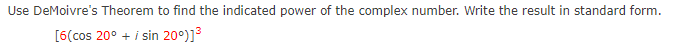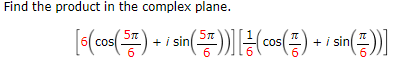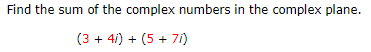Home / Expert Answers / Precalculus / use-demoivre-39-s-theorem-to-find-the-indicated-power-of-the-complex-number-write-the-result-in-st-pa178

# (Solved): Use DeMoivre's Theorem to find the indicated power of the complex number. Write the result in st ...Use DeMoivre's Theorem to find the indicated power of the complex number. Write the result in standard form. $\left[6\left(\cos 20^{\circ}+i \sin 20^{\circ}\right)\right]^{3}$ Find the product in the complex plane. $\left[6\left(\cos \left(\frac{5 \pi}{6}\right)+i \sin \left(\frac{5 \pi}{6}\right)\right)\right]\left[\frac{1}{6}\left(\cos \left(\frac{\pi}{6}\right)+i \sin \left(\frac{\pi}{6}\right)\right)\right]$ Find the sum of the complex numbers in the complex plane. $(3+4 i)+(5+7 i)$

We have an Answer from Expert